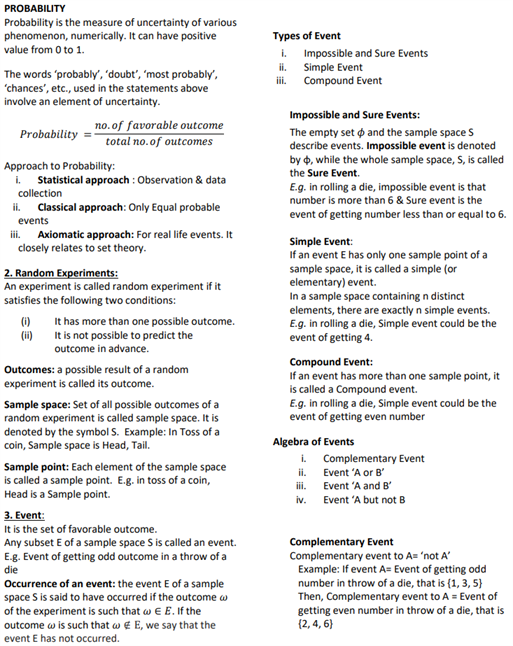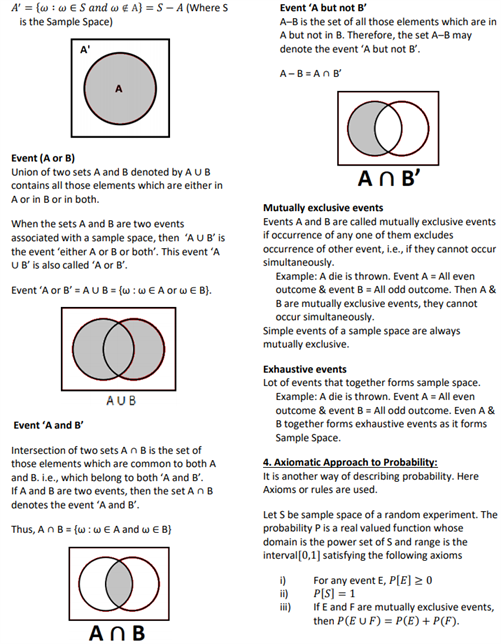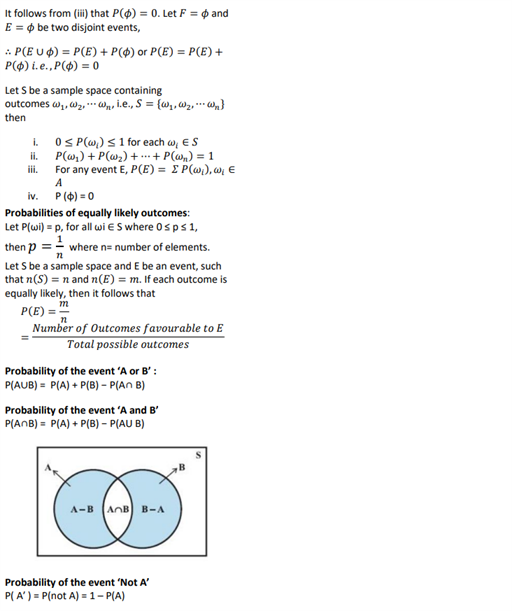# Revision Notes For CBSE Class 11 Maths Chapter 16 Probability

The class 11 Maths Revision Notes Chapter 16 Probability is one of the important chapters when it comes to understanding the basics of this chapter. We all are aware of the definition of probability etc which goes:

• Probability is the measure of uncertainty of various phenomenon, numerically. It can have a positive value from 0 to 1. The words ‘probably’, ‘doubt’, ‘most probably’, ‘chances’, etc., used in the statements above involve an element of uncertainty.
• Single Event: If an event E has only one sample point of a sample space, it is called a simple (or elementary) event. In a sample space containing n distinct elements, there are exactly n simple events.

E.g. in rolling a die, Simple event could be the event of getting 4.

In this notes, students will get to know what are random experiments, event, probabilities of equally likely outcomes etc. Preparing from this notes the important topics such as exclusive events and exhaustive events will fetch a good score in exams.

Check out the maths revision notes for class 11 chapter 16 Probability here.